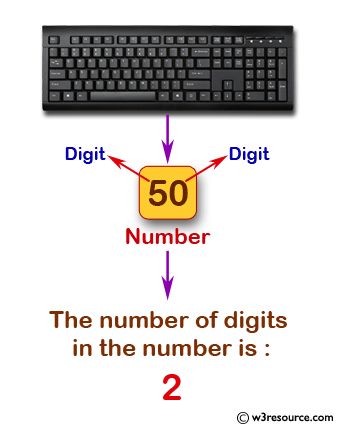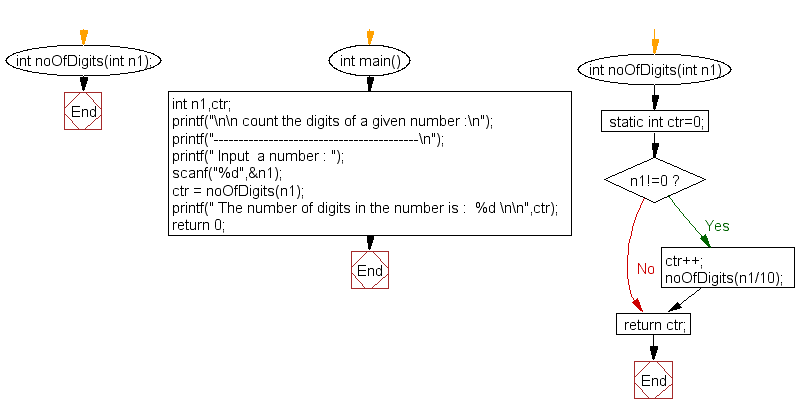﻿ C Program: Count the digits of a given number - w3resource

# C Exercises: Count the digits of a given number

## C Recursion: Exercise-5 with Solution

Write a program in C to count the digits of a given number using recursion.

Pictorial Presentation:Sample Solution:

C Code:

``````#include<stdio.h>

int noOfDigits(int n1);
int main()
{
int n1,ctr;
printf("\n\n count the digits of a given number :\n");
printf("-----------------------------------------\n");
printf(" Input  a number : ");
scanf("%d",&n1);

ctr = noOfDigits(n1);

printf(" The number of digits in the number is :  %d \n\n",ctr);
return 0;
}

int noOfDigits(int n1)
{
static int ctr=0;

if(n1!=0)
{
ctr++;
noOfDigits(n1/10);
}

return ctr;
}
```
```

Sample Output:

``` count the digits of a given number :
-----------------------------------------
Input  a number : 50
The number of digits in the number is :  2
```

Explanation:

```int noOfDigits(int n1)
{
static int ctr=0;

if(n1!=0)
{
ctr++;
noOfDigits(n1/10);
}

return ctr;
}
```

The function 'noOfDigits()' takes an integer as input and recursively counts the number of digits in the integer until the integer becomes 0. It uses a static variable ctr to keep track of the number of digits. At each recursive call, the function divides the input integer by 10 to remove the last digit and increments the ctr variable. When the input integer becomes 0, the function returns the value of ctr, which is the number of digits in the original integer.

Time complexity and space complexity:

The time complexity of the 'noOfDigits()' function is O(log n), where n is the input integer. This is because the function divides the input integer by 10 at each recursive call, so the number of recursive calls required is proportional to the number of digits in the input integer, which is log10(n).

The space complexity of the function is O(log n) as well, because the function uses a single static variable 'ctr' to keep track of the number of digits, and the maximum number of recursive calls required is log10(n).

Flowchart:C Programming Code Editor:

Have another way to solve this solution? Contribute your code (and comments) through Disqus.

What is the difficulty level of this exercise?

Test your Programming skills with w3resource's quiz.

﻿

## C Programming: Tips of the Day

__FILE__ macro shows full path

```#include <string.h>

#define __FILENAME__ (strrchr(__FILE__, '/') ? strrchr(__FILE__, '/') + 1 : __FILE__)
For Windows use '\\' instead of '/'.
```

Ref : https://bit.ly/3iEWRoT

We are closing our Disqus commenting system for some maintenanace issues. You may write to us at reach[at]yahoo[dot]com or visit us at Facebook